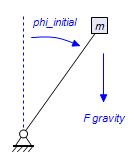﻿ 20-sim webhelp > Library > Iconic Diagrams > Mechanical > Rotation > Components > Unbalance

# Unbalance

## Library

Iconic Diagrams\Mechanical\Rotation\Components

## Use

Domains: Continuous. Size: 1-D. Kind: Iconic Diagrams (Rotation).

## Description

This model represents an unbalanced mass with an offset r to the axis of rotation and a starting angle phi_initial.The mass will act as an inertia J with a gravity induced disturbance torque:

J = r^2 * m;

alpha = (p.T + m*r*g_n*sin(phi))/ J;

p.omega = int (alpha);

phi = int (p.omega, phi_initial);

The element has a preferred angular velocity out causality. The corresponding constitutive equations then contain an integration. The element can also have the non-preferred torque out causality. The constitutive equations then contain a derivation. The model has only one rotation port p defined. Because any number of connections can be made, successive ports are named p1, p2, p3 etc. 20-sim will automatically create equations such that the resulting torque p.T is equal to the sum of the torques of all connected ports p1 .. pn and that the angular velocities of all connected ports is equal to p.omega.

p.T = sum(p1.T, p2.T, ....)

p.omega = p1.omega = p2.omega = ....

## Interface

Ports

Description

p[any]

Any number of connections can be made (Rotation).

## Causality

preferred angular velocity out

An torque out causality results in a derivative constitutive equation.

## Variables

J

phi

alpha

moment of inertia [kgm^2]

## Parameters

m

r

mass [kg]

distance of mass from center line of rotation [m]

## Initial Values

p.omega_initial

phi_initial

The initial velocity of the inertia [rad/s].

The starting angle of the mass [rad].